# AP Computer Science A : Comparing Run Times

## Example Questions

### Example Question #4 : Algorithm Analysis

Consider the following code: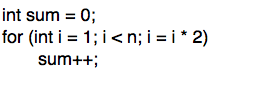What is the run-time of the code (in Big-O notation?)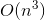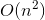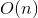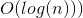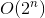Explanation:

The run-time can best be analyzed by breaking the code down by line. The line iterating the value of sum is performed in constant time, or O(1). The "for" loop that controls this constant-time operation is initialized at i = 1, and i is iterated by multiplying by 2 until it is equal to or greater than n. Thus, this loop will run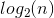times, and the run-time will be O(log(n))

### Example Question #1 : Algorithm Analysis

Consider the following code: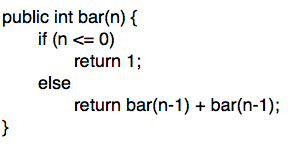What is the run time of the code (in Big-O notation?)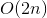Explanation:

The run time of this code is best analyzed by plugging in numbers. Take the value of bar(2) for example. bar(2) leads to two calls to bar(1), which lead to four calls to bar(0). A call to bar(4) leads to two calls to bar(3), four calls to bar(2), eight calls to bar(1), and sixteen calls to bar(0). Thus, it can be shown that the number of calls is proportional to 2^n, and that that run time is O(2^n).

### Example Question #6 : Algorithm Analysis

Consider the following code: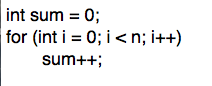What is the expected runtime of the code (in Big-O notation?)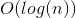Explanation:

The run-time can best be analyzed by breaking the code down by line. The line iterating the value of sum is performed in constant time, or O(1). This constant time operation is performed n times in the for loop, leading to a run time that is proportional to n, or O(n).

### Example Question #7 : Algorithm Analysis

Consider the following code: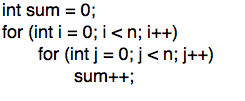What is the expected run time of the code (in Big-O notation?)Explanation:

The run time can best be analyzed by breaking the code down by line. The line iterating the value of sum is performed in constant time, or O(1). This constant time operation is performed n times in the inner "for" loop, and n times in the outer "for" loop. Thus, the run time is.

### Example Question #8 : Algorithm Analysis

Consider the following code: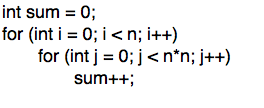What is the run time of the code (in Big-O notation)?Explanation:

The run time can best be analyzed by breaking the code down by line. The line iterating the value of sum is performed in constant time, or O(1). This constant time operation is performed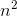times in the inner "for" loop, andtimes in the outer "for" loop. Thus, the overall run time is.

### Example Question #1 : Comparing Run Times

What is the BigO of the following function?

public void bigO(int[][] m)
{
if (m.length > 2)
{
for (int i = 0; i < m.length - 1; i++)
{
for (int j = 0; j < m[i].length; j++)
{
System.out.println(m[i][j]);
}
}
}
else
{
for (int j = 0; j < m.length; j++)
{
System.out.println(m[j]);
}
}
}

O(n)

O(1)

O(log(n))

O(n*log(n)

O(n2)

O(n2)

Explanation:

The function is O(n2) because it has a nested loop of size 2, with no extras thrown in that could possibly add on a log(n). A good rule of thumb is this: if there are nested loops, then the BigO is usually O(nm), with m being the levels of loops in the longest part.

### Example Question #10 : Algorithm Analysis

Which is more efficient (i.e. Lower Big O)?

1)

arr = [1, 2, 3, 4, 5, 6, 7, 8]

arr2 = [[1,2],[3,4],[5,6], [7,8], [9,10], [10, 11]]

for (int i = 0; i < arr.length; i++) {

for (int j = i; j < arr2.length; j++) {

arr[i][j] = 0;

}

}

2)

arr = [1, 2, 3, 4, 5, 6, 7, 8]

arr2 = [[1,2],[3,4],[5,6], [7,8], [9,10], [10, 11]]

for (int i = 0; i < arr.length; i++) {

for (int j = 0; j < arr2.length; j++) {

arr[j] = 0;

}

}

2

Irrelevant

1

The Big O is equivalent

2

Explanation:

Code sample #1 relies on i in the second loop where int j = i. Since the code relies on i in the second loop, the order goes from O(N) to O(N2)

Code sample #2 has two separate loops that do not rely on each other. The first for loop loops through the array arr and the second for loop loops through the array arr2. Since the two loops are exclusive, the order is O(N)

### Example Question #1 : Comparing Run Times

Which has faster compile time, O(N), O(N2), O(N3), or O(NlogN)?

O(NlogN)

O(N)

O(N2)

O(N3)

O(N)

Explanation:

O(NlogN) is O(N) * O(logN) which is greater than O(N) alone.

O(N2) is O(N*N) which is greater than O(N).

O(N3) is O(N*N*N) which is greater than O(N).

O(N) is the smallest and therefore is the quickest to compile. Therefore, O(N) is the correct answer.

### Example Question #1 : Comparing Run Times

Which is more efficient?

a)

arr = [0, 1, 2, 3]

for (int i = 0; i < arr.length; i++) {

int j = 0;

if (j == arr[i]) {

j++;

}

}

b)

ArrayList<Integer> arL = new ArrayList<Integer>();

arL.add(0);

arL.add(1);

arL.add(2);

arL.add(3);

for (int i = 0; i < arL.size(); i++) {

int k = 0;

if (k == arL.get(i)) {

k++;

}

}

Arrays are more efficient than ArrayLists

a)

a) and b) have the same efficiency

b)

a) and b) have the same efficiency

Explanation:

The two code snippets have the same efficiency. Both operate in O(N) time. ArrayLists use arrays as their underlying data structure so access to the data is also the same.

### Example Question #3 : Comparing Run Times

True or False.

The code snippet A has a more efficient running time than code snippet B.

(A)

for (int i = 0; i < 25; i++) {

for (int j = i; j < 25; j++) {

}

}

(B)

for (int i = 0; i < 25; i++ {

for (int j = 0; j < 25; j++) {

}

}

False

True